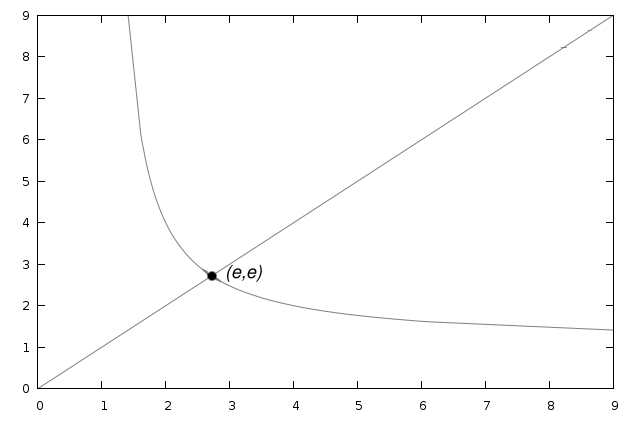#e

e, also known as Euler's number (not to be confused with Euler's constant, which is something different) is one of the fundamental mathematical constants, the base of the natural logarithm. Even though it is such a fundamental constant, it's possible you've never heard of it; I remember only learning about the number in grade 12, so if you didn't take (or haven't yet taken) mathematics around that level, e may be unfamiliar to you. If so (or even if not), read on.

To nine decimal places, e is equal to 2.718281828. You can see this on your scientific calculator by pressing the 1 key followed by the ex key. Just looking at the first nine decimal places, you might be tempted to think that it is a repeating decimal, but it isn't (the next five decimal places after the first nine are 45905, for example). Rather, it is an irrational, transcendental number.

One way to appreciate the significance of e is to imagine a very generous bank that gives 100% interest on deposits. Let's say you deposit \$1. If they compute interest yearly, after one year you will have \$(1 × (1 + 1)) = \$2. What if they compute interest twice a year? Well, after 6 months you will earn 50% interest on the \$1, giving you \$1.50. At the end of the year you will earn 50% interest, but since you now have \$1.50 in the bank, the interest accruing will be \$0.75, giving you a total of \$2.25. Numerically, the calculation would be as follows:

1 × (1 + 0.5)(1 + 0.5) = 1 ×(1 + ½)² = 2.25

If they compute interest three times per year, the amount of money that you will have in the bank after a year will be 1 ×(1 + ⅓)³ = \$2.37... , and, in general, if they compute interest n times per year, the amount you will have in the bank after one year will be (1 + 1n)n. Now, as n gets very large, this expression does not increase without bound. For example, if n = 50, you'll have \$2.691588... in your account after one year, while if n = 500, you'll have \$2.715569... in your account, less than \$0.03 more. If n = 1,000,000, you'll have \$2.71828..., less than three-tenths of a cent more. You'll also notice that this number is e to five decimal places. If you compounded interest continuously, there would be exctly e dollars in your account at the end of the year.

You might think that the preceding example is ridiculous and contrived; after all, what bank gives 100% interest? However, if you think about it, a rate of growth that is continuously equal to the amount that's growing describes perfectly the manner in which populations of any organism grow if not checked. For example, if you had a population of bacteria whose daily rate of growth was equal to the population of the colony, and there were 100 bacteria to begin with, the next day there would be 100e organisms, the day after that 100e² organisms, the day after that 100e³ organisms, and, in general, on day n there would be 100en organisms. e is useful for describing exponential growth.

If you're familiar with calculus, a function that grows at a rate equal to its current value would have a derivative equal to itself, and the derivative of ex is, in fact, ex. This is the only function that is its own derivative.

Here's another, less traditional, illustration of the significance of the number e. Consider the graph of the relation xy = yx. This graph will consist of two pieces. First, the relation will be satisfied by all points where x = y. Second, there are other points that also satisfy the relationship. For example, 42 = 24, so (2,4) and (4,2) will be on the graph. This second piece will probably be some sort of curve. Where do the two pieces meet?You guessed it, they meet at (e, e).

Here's a way to calculate the value of e using an infinite series:

e = 10! + 11! + 12! + 13! + 14! + 15! + 16! + ...

Because factorials grow very large very quickly, this sum converges to e quite quickly; after 11 terms, the value is 2.7182818..., correct to 7 decimal places.